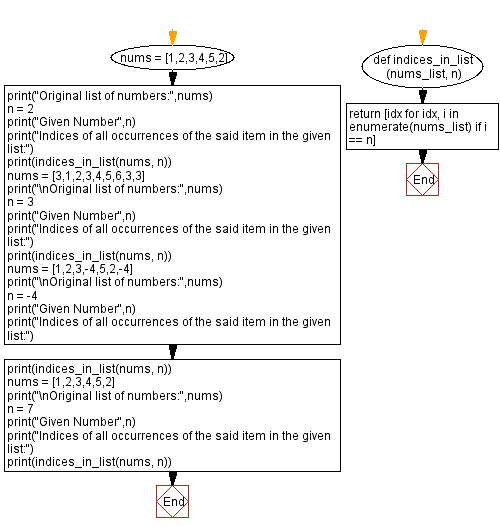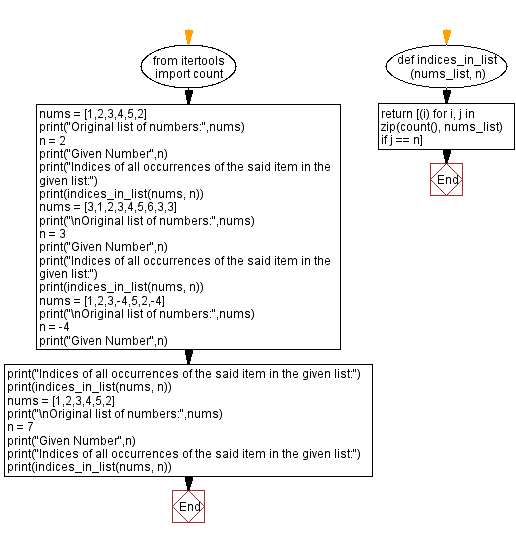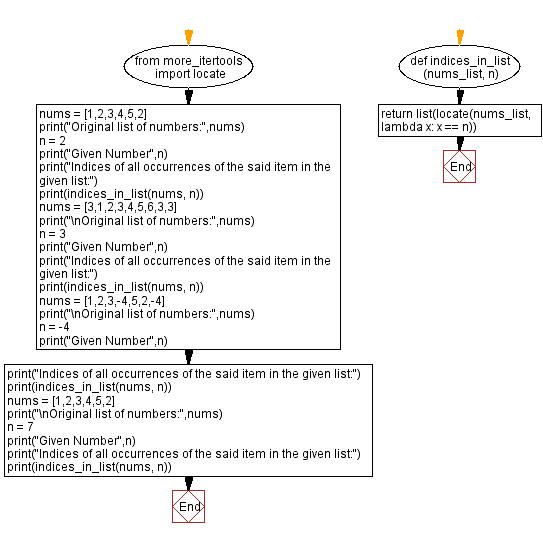﻿ Python: Find the indices of all occurrences of a given item in a given list - w3resource# Python: Find the indices of all occurrences of a given item in a given list

## Python Basic - 1: Exercise-109 with Solution

Write a Python program find the indices of all occurrences of a given item in a given list.

Sample Solution-1:

Python Code:

``````def indices_in_list(nums_list, n):
return [idx for idx, i in enumerate(nums_list) if i == n]
nums = [1,2,3,4,5,2]
print("Original list of numbers:",nums)
n = 2
print("Given Number",n)
print("Indices of all occurrences of the said item in the given list:")
print(indices_in_list(nums, n))
nums = [3,1,2,3,4,5,6,3,3]
print("\nOriginal list of numbers:",nums)
n = 3
print("Given Number",n)
print("Indices of all occurrences of the said item in the given list:")
print(indices_in_list(nums, n))
nums = [1,2,3,-4,5,2,-4]
print("\nOriginal list of numbers:",nums)
n = -4
print("Given Number",n)
print("Indices of all occurrences of the said item in the given list:")
print(indices_in_list(nums, n))
nums = [1,2,3,4,5,2]
print("\nOriginal list of numbers:",nums)
n = 7
print("Given Number",n)
print("Indices of all occurrences of the said item in the given list:")
print(indices_in_list(nums, n))
``````

Sample Output:

```Original list of numbers: [1, 2, 3, 4, 5, 2]
Given Number 2
Indices of all occurrences of the said item in the given list:
[1, 5]

Original list of numbers: [3, 1, 2, 3, 4, 5, 6, 3, 3]
Given Number 3
Indices of all occurrences of the said item in the given list:
[0, 3, 7, 8]

Original list of numbers: [1, 2, 3, -4, 5, 2, -4]
Given Number -4
Indices of all occurrences of the said item in the given list:
[3, 6]

Original list of numbers: [1, 2, 3, 4, 5, 2]
Given Number 7
Indices of all occurrences of the said item in the given list:
[]
```

Pictorial Presentation:Flowchart:Sample Solution-2:

Python Code:

``````from itertools import count
def indices_in_list(nums_list, n):
return [(i) for i, j in zip(count(), nums_list) if j == n]
nums = [1,2,3,4,5,2]
print("Original list of numbers:",nums)
n = 2
print("Given Number",n)
print("Indices of all occurrences of the said item in the given list:")
print(indices_in_list(nums, n))
nums = [3,1,2,3,4,5,6,3,3]
print("\nOriginal list of numbers:",nums)
n = 3
print("Given Number",n)
print("Indices of all occurrences of the said item in the given list:")
print(indices_in_list(nums, n))
nums = [1,2,3,-4,5,2,-4]
print("\nOriginal list of numbers:",nums)
n = -4
print("Given Number",n)
print("Indices of all occurrences of the said item in the given list:")
print(indices_in_list(nums, n))
nums = [1,2,3,4,5,2]
print("\nOriginal list of numbers:",nums)
n = 7
print("Given Number",n)
print("Indices of all occurrences of the said item in the given list:")
print(indices_in_list(nums, n))
``````

Sample Output:

```Original list of numbers: [1, 2, 3, 4, 5, 2]
Given Number 2
Indices of all occurrences of the said item in the given list:
[1, 5]

Original list of numbers: [3, 1, 2, 3, 4, 5, 6, 3, 3]
Given Number 3
Indices of all occurrences of the said item in the given list:
[0, 3, 7, 8]

Original list of numbers: [1, 2, 3, -4, 5, 2, -4]
Given Number -4
Indices of all occurrences of the said item in the given list:
[3, 6]

Original list of numbers: [1, 2, 3, 4, 5, 2]
Given Number 7
Indices of all occurrences of the said item in the given list:
[]
```

Flowchart:Sample Solution-3:

Python Code:

``````from more_itertools import locate
def indices_in_list(nums_list, n):
return list(locate(nums_list, lambda x: x == n))
nums = [1,2,3,4,5,2]
print("Original list of numbers:",nums)
n = 2
print("Given Number",n)
print("Indices of all occurrences of the said item in the given list:")
print(indices_in_list(nums, n))
nums = [3,1,2,3,4,5,6,3,3]
print("\nOriginal list of numbers:",nums)
n = 3
print("Given Number",n)
print("Indices of all occurrences of the said item in the given list:")
print(indices_in_list(nums, n))
nums = [1,2,3,-4,5,2,-4]
print("\nOriginal list of numbers:",nums)
n = -4
print("Given Number",n)
print("Indices of all occurrences of the said item in the given list:")
print(indices_in_list(nums, n))
nums = [1,2,3,4,5,2]
print("\nOriginal list of numbers:",nums)
n = 7
print("Given Number",n)
print("Indices of all occurrences of the said item in the given list:")
print(indices_in_list(nums, n))
``````

Sample Output:

```Original list of numbers: [1, 2, 3, 4, 5, 2]
Given Number 2
Indices of all occurrences of the said item in the given list:
[1, 5]
Original list of numbers: [3, 1, 2, 3, 4, 5, 6, 3, 3]
Given Number 3
Indices of all occurrences of the said item in the given list:
[0, 3, 7, 8]
Original list of numbers: [1, 2, 3, -4, 5, 2, -4]
Given Number -4
Indices of all occurrences of the said item in the given list:
[3, 6]
Original list of numbers: [1, 2, 3, 4, 5, 2]
Given Number 7
Indices of all occurrences of the said item in the given list:
[]
```

Flowchart:Python Code Editor:

Have another way to solve this solution? Contribute your code (and comments) through Disqus.

What is the difficulty level of this exercise?

Test your Programming skills with w3resource's quiz.

﻿

## Python: Tips of the Day

Iterating over dictionaries using 'for' loops:

I am a bit puzzled by the following code: d = {'x': 1, 'y': 2, 'z': 3} for key in d: print key, 'corresponds to', d[key] What I don't understand is the key portion. How does Python recognize ...

key is just a variable name.

```for key in d:
```

For Python 3.x:

```for key, value in d.items():
```

For Python 2.x:

```for key, value in d.iteritems():
```

To test for yourself, change the word key to poop.

In Python 3.x, iteritems() was replaced with simply items(), which returns a set-like view backed by the dict, like iteritems() but even better. This is also available in 2.7 as viewitems().

The operation items() will work for both 2 and 3, but in 2 it will return a list of the dictionary's (key, value) pairs, which will not reflect changes to the dict that happen after the items() call. If you want the 2.x behavior in 3.x, you can call list(d.items()).

Ref: https://bit.ly/37dm0Qo